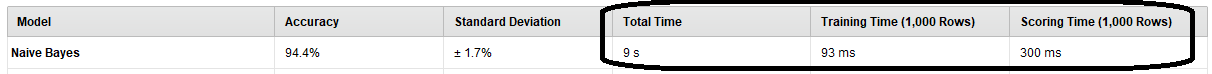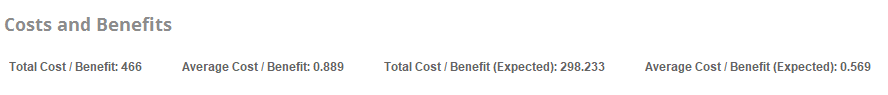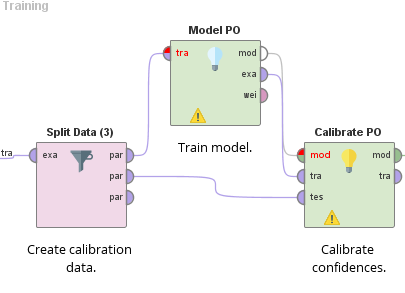# Questions on RapidMiner 9.4 Beta new releases

Moderator, RapidMiner Certified Analyst, Member Posts: 1,153Unicorn
Dear all,

I played a little bit with RapidMiner 9.4 Beta and I have several questions :

1. Is there any reason why the calculated times (scoring time, training time etc.) are not the same :
- in the results of AutoModel ("Overview" panel)AND
- in the "Results" of the model (after generating and executing the process) :NB : FYI, these results are obtained with the Naives Bayes model with the "Titanic" dataset.

2. In previous RM releases, the Performances (cost) operator calculated the misclassification cost for classification problems, which is understandable.
Now there are 4 notions displayed in the results of AutoModel. Can you explain these 4 notions :3. Can you explain further the function performed by the Rescale Confidences Logistic (Calibrate PO) operator ? (There is no description in the "Help" menu of this operator)...Thanks you for your listening,

Regards,

Lionel

Tagged:

## Best Answer

• Administrator, Moderator, Employee, RapidMiner Certified Analyst, RapidMiner Certified Expert, Community Manager, RMResearcher, Member, University Professor Posts: 1,751RM Founder
edited July 2019 Solution Accepted
Thanks for testing the Beta and for your questions.  Let me comment on them one by one:

1) different scoring times
Well, the reason is simply that if you run the process again from the designer, it is a new and different run and so you will get different results as well.  If you run the process again, you will again get somewhat different numbers.  In general, running a process in Auto Model will take a bit more time simply because RapidMiner is doing more things in parallel (creating visualizations, adding them to the UI etc.).  While this does not directly affect the process, it takes away resources from your computer which slows down the process a bit.  If you run it from the designer (and if you do nothing else ;-)), your computer can focus on the process calculation alone which typically makes it a bit faster.  But there may be other things going on in the background (like cleaning up memory or indexing your repositories etc.) so this does not always have to be the case as well...
To summarize: the exact numbers shown in Auto Model actually matter a bit less and are more directional. It is really the order of magnitude and the ranking of model times which is more interesting (in the spirit of "those models are roughly equally fast" or "this model is MUCH slower".  Hope this makes sense.

2) costs in performance results
We have introduced cost-sensitive learning (in fact: scoring) in Auto Model.  You can define the costs for different misclassifications (as well as the benefits / gains for correct predictions) in the third step of Auto Model (Prepare Target).  Those costs are then used to calculate the outcome in the performance results.  Let's go through all of them:
• The total cost / benefit is going through all rows in the validation set and sums up the costs / gains gained from each row.  So if your prediction is A and it actually is class A, you add the benefits for this (coming from the cost matrix, see above) to the total cost.  And if the your prediction is A but it actually is class B, you look up the costs for this misclassification in the cost matrix and subtract it from the total cost / benefit number.  If your cost matrix is actually \$\$\$ values, the total cost / benefits would then be the total costs / gains in \$\$\$ which you would have been achieving on your validation data set.
• The average cost / benefit is the total cost / benefit from above divided by the number of rows in your validation data.  It shows you how much \$\$\$ are generated (or lost) with each single prediction.
• The total cost / benefit (expected) number is taking the prediction confidences into account. If you look into the Predictions tab in the result, you will notice a new column called "cost".  This column contains the expected costs / benefits from each row.  We get this by multiplying all the costs / benefits from the cost matrix with the probabilities for the different outcomes.  So if your confidence for B is 0.8, but it truly is class A, you will use 80% of the misclassification cost for this row and 20% of the benefits for correctly predicting class A (if those are the only two classes).  The expected cost is what the cost-sensitive scoring algorithm is actually optimizing for, which is why we show it here.  But from a business perspective you obviously will care more about the total cost above.
• The average cost / benefit (expected) is the expected total divided by the number of rows in the validation data just like above.
3) confidence calibration
Yes, sorry, that documentation was lost in the first Beta release.  It has been added again in the Beta2 already.  The basic idea is to apply a Platt scaling on the output of a model.  This was already an operator in RapidMiner, but the new operator is more robust than the other one plus it also works for multiclass problems.
This has been added for two reasons:
1. cost-sensitive scoring requires proper probabilities (see above) and most confidence values are not. Platt scaling takes the confidence values from a model on a calibration set together with the true labels of this set and learns a logistic regression model for each class with the confidences as input and the true classes as label.  This will rescale the confidences to use more of the full 0 to 1 spectrum with 0.5 as a natural cutoff threshold.  Without that, cost-sensitive learning does not really work well.  As I said, some models are not really needing this (like logistic regression), but others really do (like SVM and FLM).  For consistency reasons we perform it everywhere though.
2. confidence values sometimes are skewed and do not match human expectations, especially for highly imbalanced data sets.  So a nice side effect of the calibration is that the confidences now act more like true probabilities and therefore what humans would expect.
If you want to learn more about this, just look for confidence calibration and Platt scaling online and you will find a ton of resources.
Hope this helps,
Ingo

## Answers

• Moderator, RapidMiner Certified Analyst, Member Posts: 1,153Unicorn
Hi @IngoRM,

That's much clearer with your explanations !
Thanks you for spending time answering these questions.

Regards,

Lionel
Sign In or Register to comment.